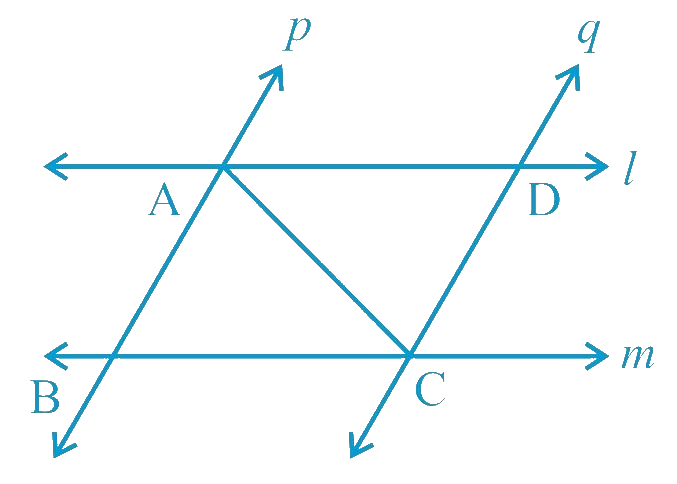# Ex.7.1 Q4 Triangles Solution - NCERT Maths Class 9

## Question

$$l$$ and $$m$$ are two parallel lines intersected by another pair of parallel lines $$p$$ and $$q$$ (see the given figure). Show that $$\Delta ABC \cong \Delta CDA$$.

Video Solution
Triangles
Ex 7.1 | Question 4

## Text Solution

What is Known?

$$l\parallel m\,\,\,and\,\,\,\,p\parallel q\,$$

To prove:

$$\Delta {\text{ABC}} \cong \Delta {\text{CDA}}{\text{.}}$$

Reasoning:

We can show both the triangles congruent by using ASA congruency criterionSteps:

In $$\Delta ABC$$ and $$\Delta CDA$$,

\begin{align}&\angle BAC \,\text{and}\, \angle DCA \\&\text{(Alternate interior angles, as}(p \|q)\\\\AC &= CA \text{(Common)}\\&\angle BAC \text{and} \angle DCA \\&\text{(Alternate interior angles, as} (l \|m)\\\\&\therefore\,\, \Delta ABC \cong \Delta CDA \\&\text{(By ASA congruence rule)}\end{align}

Video Solution
Triangles
Ex 7.1 | Question 4
Learn from the best math teachers and top your exams

• Live one on one classroom and doubt clearing
• Practice worksheets in and after class for conceptual clarity
• Personalized curriculum to keep up with school